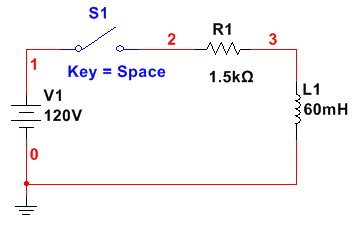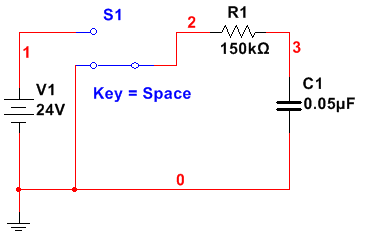# rc and l r time constants

RC and L/R Circuits

1. Consider the series L/R circuit below:
1. What is the time constant of the circuit with S1 closed?
2. What is the eventual steady-state current with S1 closed?
3. What is the value of the circuit current at the first instant S1 is closed? (t = 0s)
4. What is the value of the circuit current exactly one time constant after S1 is closed?
5. How long after S1 closes will it take before the circuit current reaches its steady-state value?
2. For the same circuit, assume that the switch S1 has been closed for more than five L/R time constants. If a 1MΩ resistor is placed across the terminals of the switch, calculate:
1. The approximate time constant of the circuit with S1 open.
2. The peak inductor voltage VL, when S1 is opened.
3. The di/dt value the instant S1 is opened.
4. How long it takes for the current to decay to zero after S1 is opened (approximately).

Series L/R Circuit:1. Consider the series RC circuit below:
1. Assume C1 is completely discharged with S1 in the position shown. If S1 is moved to the top position, how long will it take for the capacitor voltage to reach
1. 3V
2. 6V
3. 15V
4. 20V
2. Assume that C is completely discharged with S1 in the position shown. If S1 is moved to the top position, how much is the resistor voltage at the following time intervals?
1. t = 0 s
2. t = 4.5 ms
3. t = 10 ms
4. t = 15 ms
5. t = 25 ms
3. Assume that C is fully charged with S1 in the top position. If S1 is moved to the bottom position (as shown), how long with it take the capacitor to discharge to:
1. 4 V
2. 8 V
3. 12 V
4. 18 V

Series RC Circuit:Submit all calculations in a document.docx, or an equivalent word processing file extension.Must Show All Work